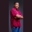Related Tags

perimeter
circle
scala
class
communitycreator

# How to get the perimeter of a circle in ScalaTheodore Kelechukwu Onyejiaku

### Overview

The perimeter of a circle is the distance around a closed figure, which in this case is a circle. It is also known as the circumference. It is the product of 2, pi, and the circle's radius.

All we need to do to find this value is to create a constant called pi using the val keyword. Next, we multiply this with other values.

### Syntax

2 * PI * radius
Syntax of perimeter of a circle in scala

### Parameters

• PI: This is pi in mathematics. Its value is 3.14.
• radius: This is the radius specified.

### Return value

The value returned is a float, which is the total distance around the circle.

### Example

// import the math module
import scala.math._

object Main extends App {
// create a PI value
val PI = Math.PI

// get the perimeter of cricles
var per1 = 2 * PI * rad1
var per2 = 2 * PI * rad2
var per3 = 2 * PI * rad3
var per4 = 2 * PI * rad4

// print the perimeters
}
Get the perimeter of a circle in Scala

### Explanation

• Line 2: We import the Math module.
• Line 6: We create a PI constant value.
• Lines 9–12: We create some radius values.
• Lines 15–18: We get the perimeter of circles with the radius created.
• Lines 21–24: We print the perimeters.

RELATED TAGS

perimeter
circle
scala
class
communitycreator

CONTRIBUTORTheodore Kelechukwu Onyejiaku
RELATED COURSES

View all Courses

Keep Exploring

Learn in-demand tech skills in half the time